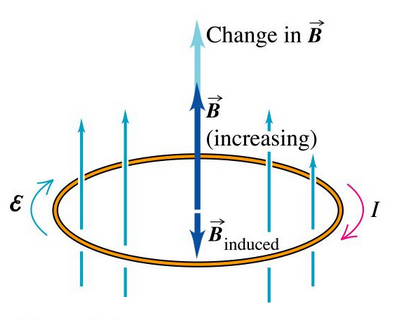# Force Acting On A Charge Moving A Magnetic Field

## Magnetism of Class 10

### ELECTROMAGNET

An electromagnet is a temporary strong magnet and is just as a solenoid with its winding on a soft iron core.

An electromagnet consists of a soft iron core AB placed inside a solenoid. The current in the solenoid can be adjusted with a rheostat Rh in the circuit having a battery and a key, K as shown in figure. An electromagnet acquires the magnetic properties only when an electric current is passed through the solenoid. Once the current is switched off, it almost loses its magnetic properties as the retentivity (the ability to retain magnetism) of soft iron is very low.

The strength of the electromagnet depends upon:

•  Amount of current flowing.
•  Number of the circular turns of wire.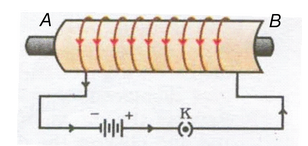In order to provide a strong magnetic field in a small region, an electromagnet is made in the U-shape. Such a magnet is called a horse-shoe magnet.

### Uses of Electromagnets

•  Electromagnets are used in electrical devices such as an electric bell, an electric fan, telegraph, an electric train, an electric motor, generator etc.
•  For lifting and transporting large masses of iron in the form of girders.
•  In medical practice, for removing pieces of iron from wounds.

### PERMANENT MAGNET

A permanent magnet is made from steel. As steel has more retentivity than iron, it does not lose its magnetism easily.

A steel bar is placed inside a solenoid AB as shown in the figure and the current is switched on and off with help of key K. On removing and testing the bar, it is found to be magnetised. It is of no use to pass the current through the solenoid for a long time because the bar will not be magnetised beyond a certain limit. On the other hand, the solenoid may be damaged due to overheating.Apart from different varieties of steel (carbon steel, chromium steel, cobalt and tungsten steel), some alloys like Alnico (aluminium, nickel and cobalt) and Nipermag (an alloy of iron, nickel, aluminum and titanium) are used to make very strong permanent magnets.

### Uses of Permanent Magnets

Permanent magnets are used in

• Electric meters (galvanometers, voltmeters, ammeters, speedometers etc.)
• Microphones and loudspeakers and
• Electric clocks.

Difference Between a Bar Magnet (for Permanent Magnet) and an Electromagnet:

 S. No. BarMagnet (permanent magnet) Electromagnet 1. The bar magnet is a permanent magnet An electromagnet is a temporary magnet. Its magnetism is only for the duration for which current passes through it, so the magnetism of an electromagnet can be switched on or switched off as desired. 2. A permanent magnet produces a comparatively weak force of attraction. An electromagnet can produce very strong magnetic force. 3. The strength of a permanent magnet cannot be changed. The strength of an electromagnet can be changed by changing the number of turns in its coil or by changing the current passing through it. 4. The (north-south) polarity of permanent magnet is fixed and cannot be changed. The polarity of an electromagnet can be changed by changing the direction of current in its coil.

Permanent magnets are usually made of alloys such as carbon-steel, chromium-steel, cobalt-steel, tungsten-steel, nipermag and alnico. Nipermag is an alloy of iron, nickel, aluminium and titanium whereas ALNICO is an alloy of aluminium, nickel and cobalt . Permanent magnets of these alloys are much more stronger than those made of ordinary steel, such strong permanent magnets are used in microphones, loudspeakers, electric clocks, ammeters, voltmeters, speedometers and many other devices.

(c) Methods of Demagnetising a Permanent Magnet :

(i) Magnet can be demagnetised by:

(A) Self-demagnetisation, if the magnet is stored without using magnetic keepers.

(B) Dropping it from a height or by rough handling.

(C) Heating or hammering the magnet.

(ii) Magnet can be demagnetised by placing it within a solenoid and passing high frequency AC through it.

### USES OF MAGNETISM IN MEDICINE:

An electric current always produces a magnetic field. Even weak ion currents that travel along the nerve cells in our body produce magnetic fields.

When we touch something, our nerves carry an electric impulse to the muscles we need to use. The impulse produces a temporary magnetic field. These fields are very weak and are one billionth of the earth's magnetic field. Heart and brain are the two main organs in the human body where the magnetic field produced is significant. The magnetic field inside the body forms the base of obtaining the images of different body parts. This is done by using a technique called Magnetic Resonance Images (MRI). Analysis of these images helps in medical diagnosis. Magnetism has thus, got important uses in medicine.

### MAGNETIC FORCE :

(a) Force on a Current-Carrying Conductor in a Magnetic Field :

Immediately after Oersted's discovery of electric currents producing magnetic fields and exerting forces on magnets, Ampere suggested that magnet must also exert equal and opposite force on a current-carrying conductor. When a current carrying conductor is kept in a magnetic field (not parallel to it), a force acts on it. This force is created due to the interaction of magnetic field of the current in the conductor and the external magnetic field on the conductor. As a result of this superposition, the resultant magnetic field on one side of conductor is weaker than on the other side. Hence the conductor experiences a resultant force in one direction.

Take a small aluminium rod AB. Suspend it horizontally by means of two connecting wires from a stand. Now, place a strong horseshoe magnet in such a way that the rod is between the two poles with the field directed upwards. If a current is now passed in the rod from B to A, we will observe that the rod gets displaced. This displacement is caused by the force acting on the current-carrying rod. The magnet exerts a force on the rod directed towards the right, with the result the rod will get deflected to the right. If we reverse the current or interchange the poles of the magnet, the deflection of the rod will reverse, indicating thereby that the direction of the force acting on it gets reversed. This shows that there is a relationship among the directions of the current, the field and the motion of the conductor.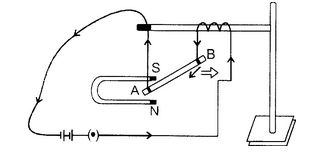### Direction of Force on Current Carrying Conductor:

The direction of force is obtained by the Fleming's left hand rule.

Fleming's left hand rule :

Stretch the forefinger, middle finger and the thumb of your left hand mutually perpendicular to each other as shown in figure. If the forefinger indicates the direction of the magnetic field and the middle finger indicates the direction of current, then the thumb will indicate the direction of motion (i.e., force) on the conductor.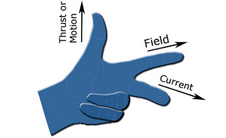Magnitude of Force:

Experimentally it is found that the magnitude of the force acting on a current carrying conductor kept in a magnetic field in direction perpendicular to it, depends on the following factors :

• The force F is directly proportional to the current flowing in the conductor, i.e. F α l.
•  The force F is directly proportional to the intensity of magnetic field, i.e. F α B.
• The force F is directly proportional to the length of the conductor (inside the magnetic field), i.e. Fα l.

Combining these we get, F = IBI or F = K I B l

Where K is constant whose value depends on the choice of units. In S.I. units K = 1 and the unit of magnetic field is tesla (T). 1 tesla is equal to 1 newton ampere-1 metre-1 or 1 weber metre-2.

Force is directly proportional to sinΘ where A is the angle between current and the direction of magnetic field. I.e., F α sinθ

Combining all we have F = BIl sinθ

Special cases:

(i) When θ = 00 or 1800, sinθ = 0 ⇒ F = 0

Force on a current - carrying conductor placed parallel or antiparallel to magnetic field is zero.

(ii) If θ = 90°, sin θ =sin 90° = 1, F= BIl is the maximum force. Force experienced by the conductor is maximum when placed perpendicular to magnetic field.

(iii) If B = 0, F = 0 i.e. the coil placed in field free area doesn't experience any force.

A moving charge in a magnetic field (direction of motion not parallel to the field direction) experiences a force called Lorentz force. Since current is due to flow of charge, therefore a conductor carrying current will experience a force.

The force acting on a current-carrying conductor placed in a magnetic field is: F=BIl

Now, if a charge Q flows in time t then the current I = Q/t . So, writing Q/t in place of I in the above equation, we get: F = B x Q x 1/t

Suppose the particle carrying the charge Q travels a length l in time t. Then the velocity v of the charged particle will be equal to I/t . Writing v in place of I/t  in the above equation, we get:

Force on moving charge, F = B x Q x v

Where B = Magnitude of magnetic field, Q = Charge on the moving particle and v = Velocity of the charged particle (in metre per second). In vector notation

When an electric current is passed through a conductor, a magnetic field is produced around the conductor. Faraday thought that as a magnetic field is produced by electric current, it should be possible to produce an electric current by the magnetic field. According to him, whenever there is a change in the magnetic lines of force associated with a conductor, an electromotive force (e.m.f.) is set up at the ends of the conductor which lasts as long as the change is taking place. This phenomenon is called electromagnetic induction.

### FORCE ACTING ON A CHARGE MOVING A MAGNETIC FIELD

We have just learnt that a current-carrying conductor experiences a force when placed in a magnetic field. But we know that current is due to charges in motion. Thus, it is clear that a charge moving in a magnetic field experiences a force, except when it is moving in a direction parallel to it.

### DIRECTION OF FORCE:

Since the direction of current is the same as that of the motion of a positive charge, the direction of force acting on it, when moving perpendicular to direction of magnetic field, is the same as that acting on a current-carrying conductor placed perpendicular to the direction of magnetic field. The direction of force is given by Fleming's left-hand rule. Obviously, the force acting on a negative charge moving in a direction perpendicular to the magnetic field is opposite to that acting on a positive charge.

### CHARACTERISTICS OF MAGNETIC FORCE:

• Magnetic force acts only on moving charges and not on stationary charges.
• No magnetic force acts on a charge if it is moving along the direction of the magnetic field.
• The direction of magnetic force is perpendicular to
• (i) the direction of velocity of the charge and
• (ii) the direction of the magnetic field.
• The magnetic force (F) depends on the charge (q), velocity (v) and the strength (B) of the magnetic field i.e. F = q v B (in case the direction of v is perpendicular to the direction of B).
• The magnetic force (F) acting on a current-carrying conductor placed perpendicular to the direction of magnetic field (B) is given by F =  l B.

where I is the current flowing in the conductor,  is its length in the magnetic field.

Wind an insulated copper wire on a wooden cylinder so as to form a solenoid coil. Connect the two ends of the coil to the centre of galvanometer. A magnet is placed along the axis of the coil.

• When the magnet is stationary, there is no deflection in the galvanometer. The pointer reads zero as shown in figure (A).
•  When the north pole of the magnet is brought near the coil, the current flows in the coil in left direction shown in the figure (B) and the galvanometer shows the deflection towards the right.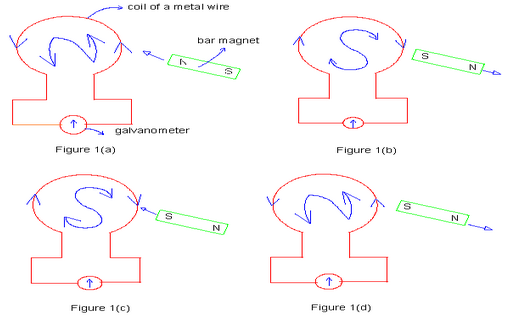• If we stop the motion of the magnet, the pointer of the galvanometer comes to the zero position as shown in figure (C). Thus the current in the coil flows so long as the magnet is moving. If the magnet is taken away from the coil, the current again flows in the coil but in the direction opposite to that shown in figure (D) and therefore the pointer of the galvanometer deflects towards the leftside.
• If south pole of the magnet is brought towards the coil, the current in the coil flows in the direction opposite to that shown in figure (E) and so the pointer of the galvanometer deflects towards the left.
•  Similar deflection is observed in the galvanometer if the magnet is kept stationary and the coil is moved.

From this experiment Faraday concluded that:

• The galvanometer shows a deflection (i.e. current flow in the coil) only when there is a relative motion between the coil and the magnet.
• The direction of deflection is reversed if the direction of motion is reversed.
• The value of the current in the coil (i.e. deflection of the pointer) is increased by:

(A) the rapid motion of the magnet or the coil.

(B) the use of a strong magnet.

(C) increasing the area and number of turns in the coil.

When the magnet and coil are relatively at rest, the total number of magnetic lines of force due to the magnet passing through the coil (i.e. the magnetic flux linked with the coil) remains constant, therefore no e.m.f. is induced in the coil and the galvanometer shows no deflection.

When there is relative motion between the coil and magnet, the magnetic flux linked with the coil changes. If the coil is moved towards the magnet, the magnetic flux through the coil increases.Due to change in magnetic flux linked with the coil, an e.m.f. is induced in the coil. This e.m.f. causes a current to flow if the circuit of the coil is closed.

### Faraday's Laws of Electromagnetic Induction :

Faraday formulated the following two laws of electromagnetic induction:

Whenever there is a change in magnetic flux linked with a conductor, an e.m.f. is induced. The induced e.m.f. lasts so long as there is a change in magnetic flux cut by the conductor.

The magnitude of the e.m.f. induced is directly proportional to the rate of change of magnetic flux cut by the conductor. If the rate of change of magnetic flux remains uniform, a steady e.m.f. is induced. If the circuit of conductor is closed, a current flows in the conductor due to the e.m.f. induced across its ends.

### Direction of Induced e.m.f:

The direction of induced e.m.f. (and hence the direction of induced current) can be obtained by any or the following rules:

(i) Fleming's right hand rule (ii) Lenz's law

### Fleming's right hand rule:

Stretch the thumb, middle finger and the forefinger of your right hand mutually perpendicular to each other as shown in figure. If the forefinger indicates the direction of the magnetic field and the thumb indicates the direction of motion of the conductor, then the middle finger will indicate the direction of induced current.

### Lenz's law:

This law gives us the direction of current induced in a circuit. According to Lenz's law, the induced current will appear in such a direction that it opposes the change (in magnetic flux) responsible for its production.

The law refers to induced currents, which means that it applies only to closed circuits. If the circuit is open, we would find the direction of induced e.m.f. For example, in figure, when the magnet is moved towards the loop, a current is induced in the loop. The induced current produces its own magnetic field with magnetic dipole moment M oriented so as to oppose the motion of the magnet. Thus the induced current must be anticlockwise as shown in figure below.